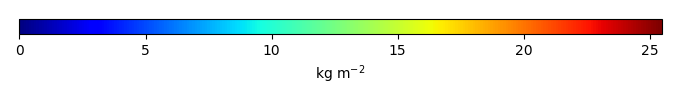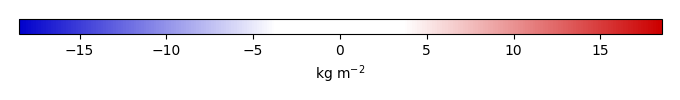# Mean State

Period Mean (original grids) [Pg]
Model Period Mean (intersection) [Pg]
Model Period Mean (complement) [Pg]
Benchmark Period Mean (intersection) [Pg]
Benchmark Period Mean (complement) [Pg]
Bias [kg m-2]
Bias Score 
Spatial Distribution Score 
Overall Score 
Benchmark [-] 30.1
CLM5PHSOFF [-] 542. 14.0 529. 29.9 0.195 -1.72 0.46 0.63 0.54
CLM5PHSON [-] 487. 22.3 465. 29.9 0.195 -0.481 0.58 0.77 0.67
Period Mean (original grids) [Pg]
Model Period Mean (intersection) [Pg]
Model Period Mean (complement) [Pg]
Benchmark Period Mean (intersection) [Pg]
Benchmark Period Mean (complement) [Pg]
Bias [kg m-2]
Bias Score 
Spatial Distribution Score 
Overall Score 
Benchmark [-] 1.44
CLM5PHSOFF [-] 11.6 4.77 6.63 1.41 0.0279 2.80 0.34 0.42 0.38
CLM5PHSON [-] 14.5 5.95 8.42 1.41 0.0279 4.18 0.21 0.47 0.34
Period Mean (original grids) [Pg]
Model Period Mean (intersection) [Pg]
Model Period Mean (complement) [Pg]
Benchmark Period Mean (intersection) [Pg]
Benchmark Period Mean (complement) [Pg]
Bias [kg m-2]
Bias Score 
Spatial Distribution Score 
Overall Score 
Benchmark [-] 0.00649
CLM5PHSOFF [-] 16.3 0.0151 15.5 0.00649 0.00 0.0419 0.44 0.27 0.35
CLM5PHSON [-] 10.2 0.0250 9.91 0.00649 0.00 0.233 0.58 0.75 0.67
Period Mean (original grids) [Pg]
Model Period Mean (intersection) [Pg]
Model Period Mean (complement) [Pg]
Benchmark Period Mean (intersection) [Pg]
Benchmark Period Mean (complement) [Pg]
Bias [kg m-2]
Bias Score 
Spatial Distribution Score 
Overall Score 
Benchmark [-] 17.4
CLM5PHSOFF [-] 7.11 5.92 0.831 17.3 0.0180 -2.78 0.49 0.62 0.55
CLM5PHSON [-] 12.0 11.6 0.244 17.3 0.0180 -1.06 0.65 0.85 0.75
Period Mean (original grids) [Pg]
Model Period Mean (intersection) [Pg]
Model Period Mean (complement) [Pg]
Benchmark Period Mean (intersection) [Pg]
Benchmark Period Mean (complement) [Pg]
Bias [kg m-2]
Bias Score 
Spatial Distribution Score 
Overall Score 
Benchmark [-] 1.52
CLM5PHSOFF [-] 32.6 1.92 30.7 1.46 0.0621 1.56 0.65 0.91 0.78
CLM5PHSON [-] 43.6 1.80 42.0 1.46 0.0621 3.72 0.65 0.90 0.77
Period Mean (original grids) [Pg]
Model Period Mean (intersection) [Pg]
Model Period Mean (complement) [Pg]
Benchmark Period Mean (intersection) [Pg]
Benchmark Period Mean (complement) [Pg]
Bias [kg m-2]
Bias Score 
Spatial Distribution Score 
Overall Score 
Benchmark [-] 9.78
CLM5PHSOFF [-] 1.45 1.30 0.251 9.71 0.0683 -2.34 0.40 0.21 0.31
CLM5PHSON [-] 3.04 2.93 0.149 9.71 0.0683 -1.73 0.48 0.41 0.45

# Temporally integrated period mean

BENCHMARK MEANMODEL MEANBIASBIAS SCORESPATIAL TAYLOR DIAGRAMMODEL COLORS# Spatially integrated regional mean

MODEL COLORS# All Models

BenchmarkCLM5PHSOFFCLM5PHSON